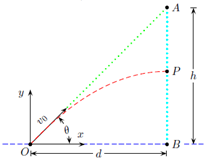Problem: Consider the setup of a gun aimed at a target (such as a monkey) as shown in the figure. The target is to be dropped from the point A at t = 0, the same moment as the gun is fired. The bullet hits the target at a point P. Let the initial speed of the bullet be v0 = 108 m/s, let the angle between the vector v0 and the horizontal (x) direction be θ = 50.9° and let AB = 90.2 m. The distance d = OB is the x-coordinate of the target. The acceleration of gravity is 9.8 m/s2 . The height BP where the collision takes place is

FREE Expert Solution
83% (399 ratings)
Problem Details

Consider the setup of a gun aimed at a target (such as a monkey) as shown in the figure. The target is to be dropped from the point A at t = 0, the same moment as the gun is fired. The bullet hits the target at a point P. Let the initial speed of the bullet be v0 = 108 m/s, let the angle between the vector v0 and the horizontal (x) direction be θ = 50.9° and let AB = 90.2 m. The distance d = OB is the x-coordinate of the target. The acceleration of gravity is 9.8 m/s2 . The height BP where the collision takes place is